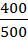# CAT 2019 Question Paper | Quants Slot 1

###### CAT Previous Year Paper | CAT Quants Questions | Question 25

Averages and Mixtures are easy topics to crack once you have had sufficient practice. The question in CAT 2019 is no different. Almost all CAT previous year paper would have a mixtures question that you can practice from.

Question 25 : A chemist mixes two liquids 1 and 2. One litre of liquid 1 weighs 1 kg and one litre of liquid 2 weighs 800 gm. If half litre of the mixture weighs 480 gm, then the percentage of liquid 1 in the mixture, in terms of volume, is

1. 70
2. 85
3. 80
4. 75

## Best CAT Coaching in Chennai

#### CAT Coaching in Chennai - CAT 2022Limited Seats Available - Register Now!

In Liquid L1 - 1L = 1000 grams
So, 1000 mL = 1000 grams
1 mL = 1 gram
x mL = x grams
In Liquid L2 - 1L = 800 grams
1000 mL = 800 grams
1mL = 0.8 grams
(500-x) mL = (500-x) x 0.8 grams
Total mass = x + 400 - 0.8x = 480 grams
0.2x = 80 grams
x = 400 grams
Therefore, Liquid 1 has 400 mL and Liquid 2 has 500 - 400 = 100 Ml
Therefore, Percentage of Liquid 1 =x 100 = 80%

The question is "A chemist mixes two liquids 1 and 2. One litre of liquid 1 weighs 1 kg and one litre of liquid 2 weighs 800 gm. If half litre of the mixture weighs 480 gm, then the percentage of liquid 1 in the mixture, in terms of volume, is "

##### Hence, the answer is 80

Choice C is the correct answer.

###### CAT Coaching in ChennaiCAT 2023

Classroom Batches Starting Now! @Gopalapuram

###### Best CAT Coaching in Chennai Introductory offer of 5000/-

Attend a Demo Class

##### Where is 2IIM located?

2IIM Online CAT Coaching
A Fermat Education Initiative,
58/16, Indira Gandhi Street,
Kaveri Rangan Nagar, Saligramam, Chennai 600 093

##### How to reach 2IIM?

Mobile: (91) 99626 48484 / 94459 38484
WhatsApp: WhatsApp Now
Email: info@2iim.com